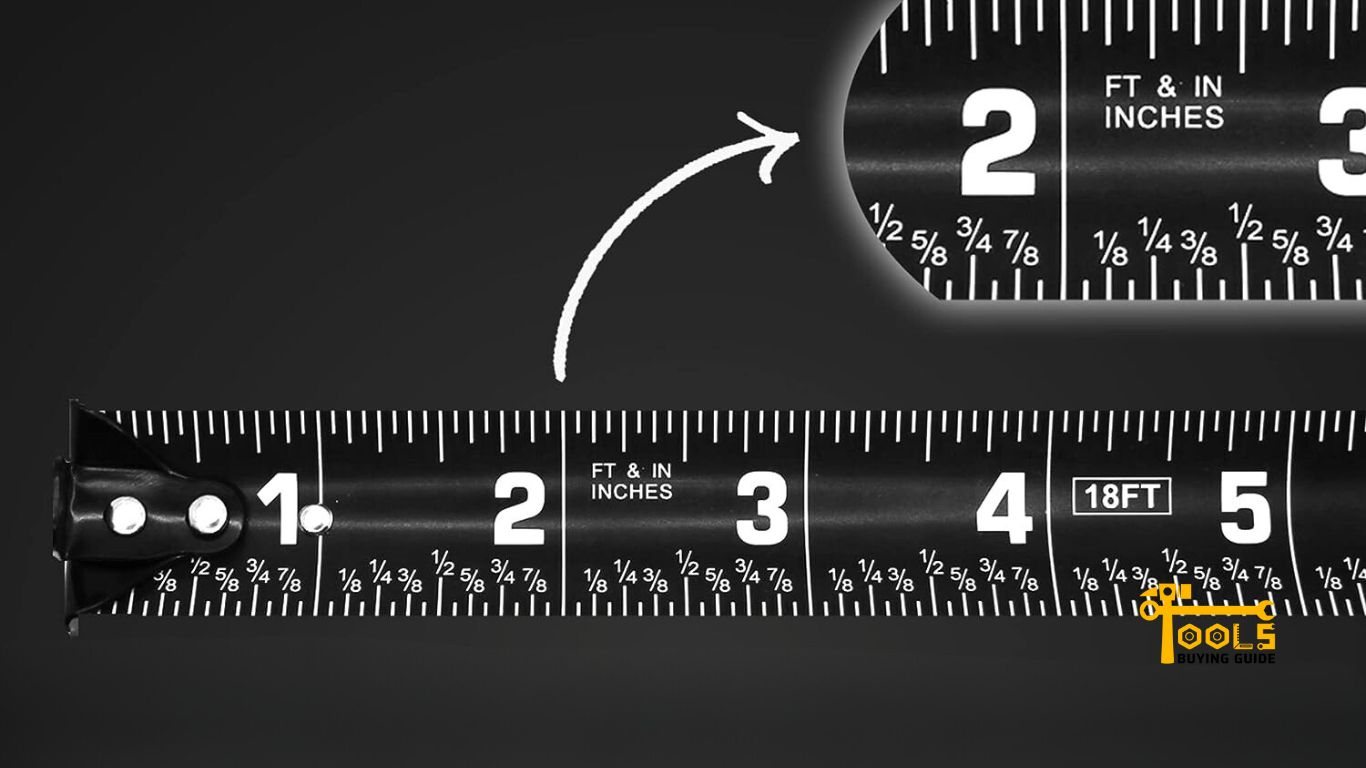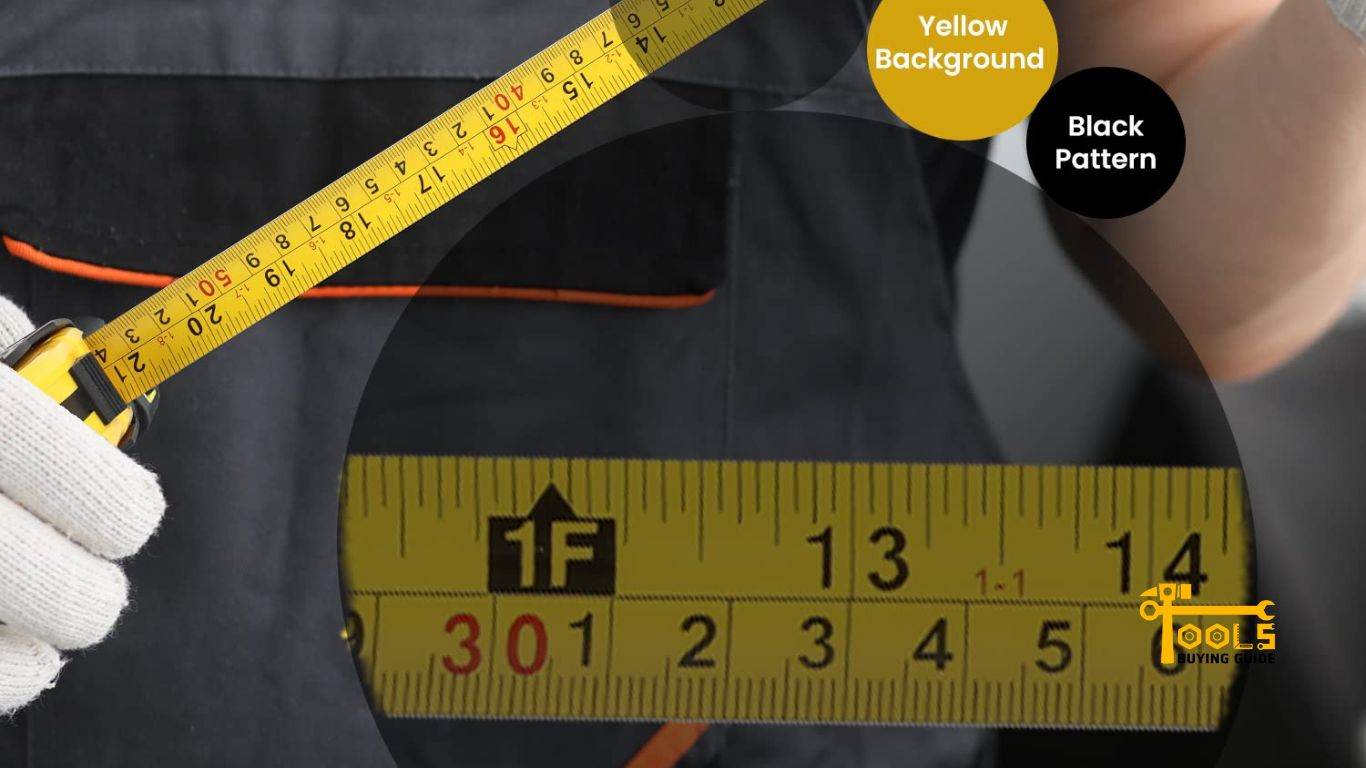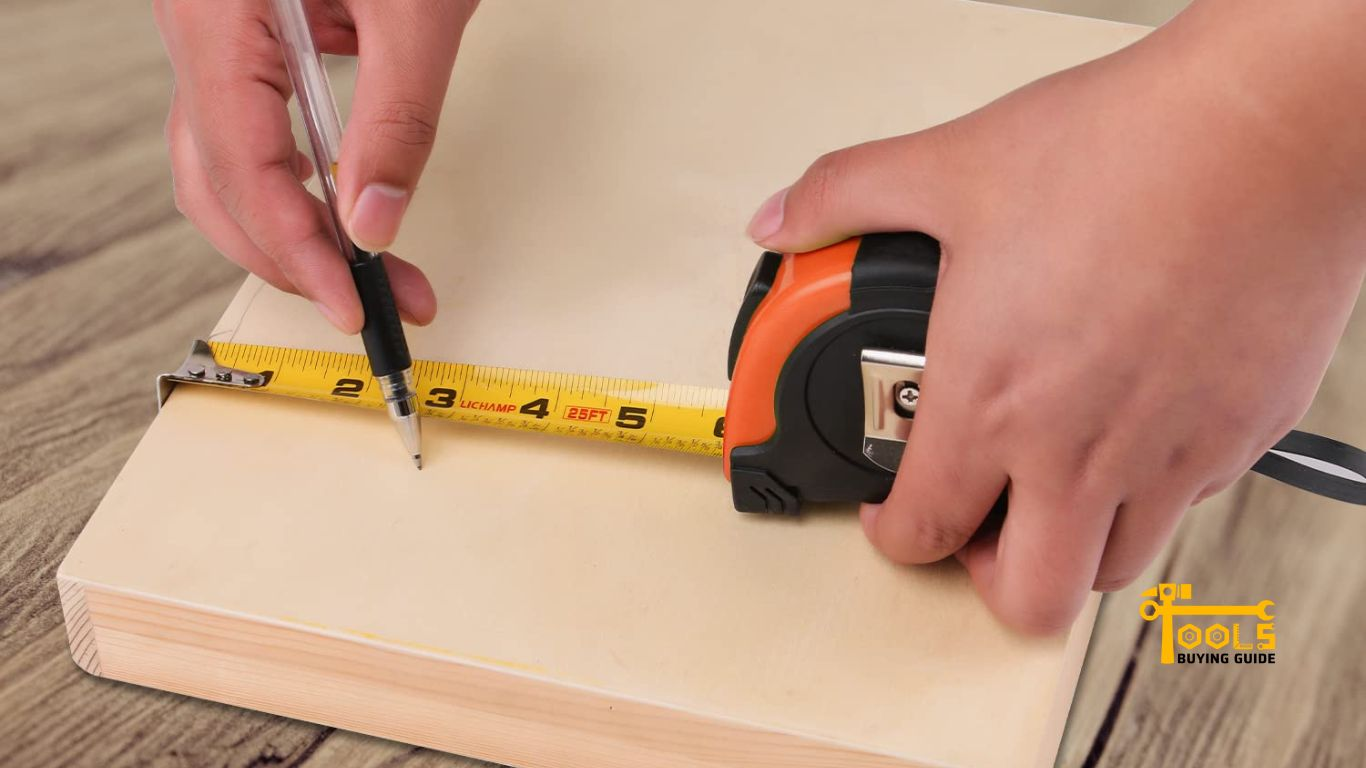# How to Read a Tape Measure Like an Expert: A Comprehensive Guide

Measuring accurately is an essential skill that every handyperson should have. In the world of DIY projects, accuracy is key. And one of the most important tools in a handyman’s arsenal is the tape measure. Mastering how to read a tape measure can help you take your DIY projects to the next level and ensure accuracy every time.

In this guide, we’ll cover the basics of tape measuring. Starting with inch and fractional markings, reading centimeters, and tips for more accurate measurements. With this guide, you’ll be a pro at reading a tape measure quickly.## How to Read a Tape Measure?

A tape measure, also called measuring tape, is a flexible type of ruler, measuring up to 25 feet. It’s made of plastic or fiberglass with inch markings on one side and metric (centimeter) markings on the other.

Tape measures have two distinctive parts – the blade or ribbon and the housing or casing. The blade is a long section with inch and metric markings. At the same time, the housing is the plastic casing that holds the blade when it’s not used. For tape measure reading, there are two main systems – imperial (inch) and metric (centimeter).

## Reading Inch Markings on the Tape Measure

Now that you understand the basics of a tape measure, let’s get into reading tape measure markings in inhces.

### Identifying whole and fractional inches

The first number on the left side of the tape measure is the length in whole inches. These are the numbers that are furthest away from the beginning. The inch markings come in 1/16th, 1/8th, and 1/4 increments. This means each inch has 16, 8, and 4 increments.

To identify fractional inches, you’ll have to look at the numbers on the right side of the tape measure. These are usually red and mark out 1/16th or 1/8th of an inch. To find 1/4th of an inch, look for a small triangle between two lines on the blade.

### Subdividing the inch into sixteenths and eighths

To get a more exact measurement, you can also subdivide the inch. To do this, you’ll need to be able to identify 1/16th and 1/8th increments.

1/16th of an inch is the smallest marking on the tape measure. The easiest way to spot them is by looking for the red numbers on the right side of the blade. The bigger numbers are 1/8th of an inch and can be found on the right side. You can identify the 1/8th mark by its two red hash marks.

### Converting decimals to inches

Sometimes you may encounter measurements that are displayed in decimal form. These numbers are easier for measuring tape inches and can be quickly converted into fractional inches on a tape measure.

To convert decimals into inches, look at the first number after the decimal point. This is how many 1/16th of an inch you have in your measurement. Then look at the second number to determine how many 1/8ths of an inch you have. You can convert any decimals up to two digits accurately using this method.

For example, if you measure 1.75 inches on your tape measure, it is equivalent to 1 3/4 inches. The first number (1) indicates that there is one full inch. The second number (7) shows that you have 7/16ths of an inch in addition to the whole inch. Finally, the last number (5) tells us we have 5/8ths of an inch.

So when all the fractions are added together, we get 1 3/4 inches.## Reading Centimeters on the Tape Measure

In addition to reading inches, you can also read centimeters on most tape measures. This is important if you’re working with metric measurements or need a more precise measurement than what is provided in inches.

### Identifying whole and fractional centimeters

On the opposite side of the tape measure, you’ll see centimeter measurements marked out in centimeters and millimeters. They work almost exactly like inch markings, except they are usually smaller and more closely spaced together. The numbers on the left side mark out the length in cm, and the numbers on the right indicate the fractional parts of a centimeter.

To measure in centimeters, look at the number on the left side. This is how many whole centimeters you have in your measurement. Then look at the number to see if there are any fractional parts of a centimeter. To identify fractions of a centimeter on measuring tape, look for the small red numbers on the right side.

### Subdividing the centimeter into millimeters

You can subdivide the centimeter into millimeters to get an even more accurate measurement. This involves looking at the small red numbers to the right of each centimeter. These represent the fractions of a centimeter and correspond to millimeters on a measuring tape. For example, one fractional part of a centimeter equals 10 millimeters.

### Converting decimals to centimeters

You can also convert decimals into centimeters on a tape measure like inches. Start by looking at the first number after the decimal point. This will tell you how many fractions of a centimeter you have. Then look at the second number to determine how many millimeters you have.

For example, if the measurement reads 12.50 centimeters, it is equivalent to 12 cm and 5 mm. The first number (12) tells us we have 12 full centimeters in our measurement. The second number (5) shows that we have 5 millimeters in addition to the full centimeters.

So when all the fractions are added together, we get 12 cm and 5 mm.

Read Also: How To Port a Chainsaw?## Tips for More Accurate Measurements

Now that you know how to read a measuring tape, here are some tips for making more accurate measurements.

### Use the tape measure in a straight line

It’s important to ensure you are using the tape measure in a straight line. Otherwise, your measurements will be off and inaccurate. So extend the blade straight line before measuring and keep it as taut as possible. This will ensure that your measurements are accurate and precise.

### Mark off sections of the measurement

If you’re measuring a long distance, keeping track of where you are on the tape measure can be difficult. So it’s helpful to mark off sections of the measurement as you go. This will make tracking where you are on the tape measure easier and ensure that your measurements don’t get off.

Finally, always double-check your measurements before cutting or drilling. This is especially important when working with wood or other materials that can easily splinter or break if cut too short. So take your time and measure twice to get an accurate reading.

### Burn an Inch for reference

Burning an inch into the material you’re working with can also be a great way to ensure accuracy. Burning tape measure marks take some guesswork out of measuring and make it easier to get a precise measurement. So if you’re doing any type of carpentry or woodworking, burning an inch for reference is always a good practice.

By following these tips, you can ensure that your measurements are always accurate and precise. Take time to learn the basics of reading a measuring tape and master the art of taking precise measurements!Is a measuring tape in cm or m?

Most measuring tapes are marked in cm and m, so you can choose to measure in metric or imperial. However, if you need to measure in specific increments, such as inches or fractions of an inch, you can purchase measuring tapes marked only with those units.

How do you read a tape measure in MM?

Look for the smallest increments on the ruler to read mm on tape measure. These will be marked in millimeters which are the metric equivalent of inches. You must count how many of these small marks are between two larger marks and then multiply that number by 10 to get an accurate measurement.

What is 4mm on a measuring tape?

4mm on a tape measure equals approximately 5/32 of an inch. To find this measurement on a ruler, look for the smallest increments, usually marked in millimeters, and then count how many are between two larger marks.

How do you read a ruler in cm and mm?

To read a ruler in cm and mm, look for the longest marks on the ruler, which indicate centimeters. Each centimeter will be divided into 10 smaller increments that represent millimeters. To get an accurate measurement, count how many of these small marks are between two larger ones and then multiply by 10 to get your total measurement in millimeters.

How thick is 4mm?

4mm on measuring tape is roughly the thickness of two sheets of paper. It is also equal to approximately 5/32 of an inch. This measurement can be found on a measuring tape for the smallest increments, usually marked in millimeters.

How many inches make 1 m?

1 meter is equal to approximately 39.37 inches. To convert your metric measurements into imperial, multiply the meters’ value by 39.37. For example, if you want to convert 4 meters into inches, multiply it by 39.37 – 4 x 39.37 = 157.48 inches.

## Conclusion

Learning how to read a tape measure is essential for any DIYer, carpenter, or handyman. With just a little practice and patience, you’ll soon be conquering projects confidently. So keep your eyes peeled for measurements and start measuring like a pro today.

With the right knowledge and know-how, you can achieve amazing results that make any project look like a masterpiece. So start practicing and become the master of measuring with a tape measure.